# 【数据结构与算法】手写HashMap的模拟实现

✨哈喽，进来的小伙伴们，你们好耶！✨

🛰️🛰️系列专栏:【数据结构与算法】

✈️✈️本篇内容:手写HashMap的模拟实现！

🚀🚀代码存放仓库gitee：Java数据结构代码存放！

⛵⛵作者简介：一名双非本科大三在读的科班Java编程小白，道阻且长，你我同行

🚋🚋给大家推荐一个超级好用的刷题网站—牛客网点击链接注册，开启刷题之路！# 一、手写HashMap

### 🍊方法一(基本类型)

🍅1、内部类准备

``````  static class Node {
public int key;
public int val;
public Node next;

public Node(int key, int val) {
this.key = key;
this.val = val;
}
}

public Node[] array;
public int usedSize;

public HashBuck() {
array = new Node;
}``````

🍎2、实现put以及头插法

``````  public void put(int key,int val) {
int index = key % array.length;
//遍历Index下标的数组，如果有相同的key那么替换
Node cur = array[index];
while (cur != null) {
if(cur.key == key) {
cur.val = val;
return;
}
cur = cur.next;
}
//进行头插法
Node node = new Node(key, val);
node.next = array[index];
array[index] = node;
usedSize++;
resize();
}
}
``````

🍏3、扩容函数resize

``````   private void resize() {
Node[] newArray = new Node[2*array.length];
for (int i = 0; i < array.length; i++) {
Node cur = array[i];
while (cur != null) {
Node curNext = cur.next;
int newIndex = cur.key % newArray.length;
//拿着cur节点 进行插入到新的位置
cur.next = newArray[newIndex];
newArray[newIndex] = cur;
cur =  curNext;
}
}
array = newArray;
}``````

🍋实验： 这里我们给出main函数代码，来测试一下我们的resize()函数是否有问题。

``````   public static void main(String[] args) {
HashBuck hashBuck = new HashBuck();
hashBuck.put(1,6);
hashBuck.put(2,7);
hashBuck.put(3,8);
hashBuck.put(4,54);
hashBuck.put(5,66);
hashBuck.put(6,234);
hashBuck.put(15,888);
System.out.println(hashBuck.get(6));
}``````

🍯这里我们给出的数组空间大小是8，那么已知负载因子是0.75，所以我们在第六个元素的位置打个断点， 来debug一下。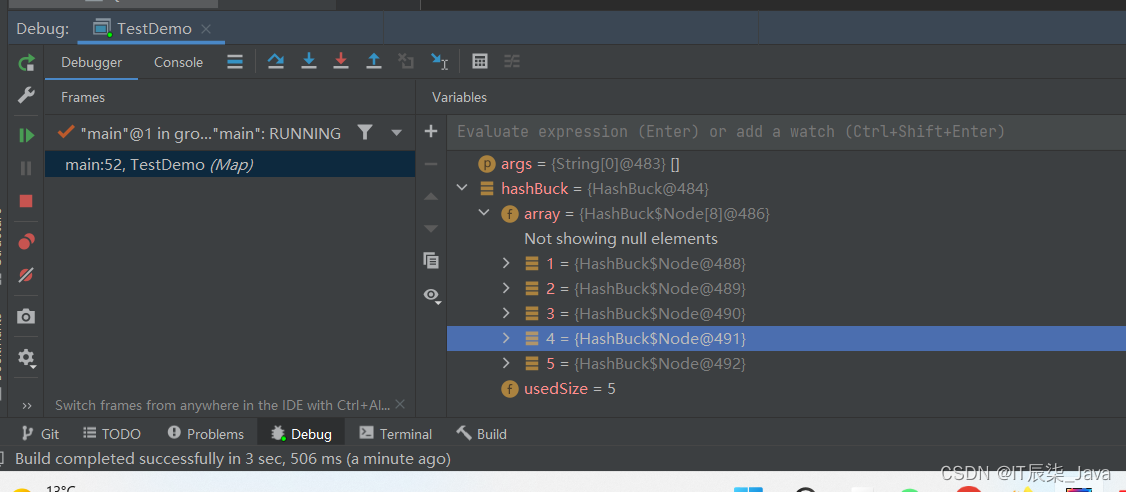🍻 我们发现，元素的存储没有问题，那么点击上面那个蓝色往右下角偏移的箭头，代码会向下执行。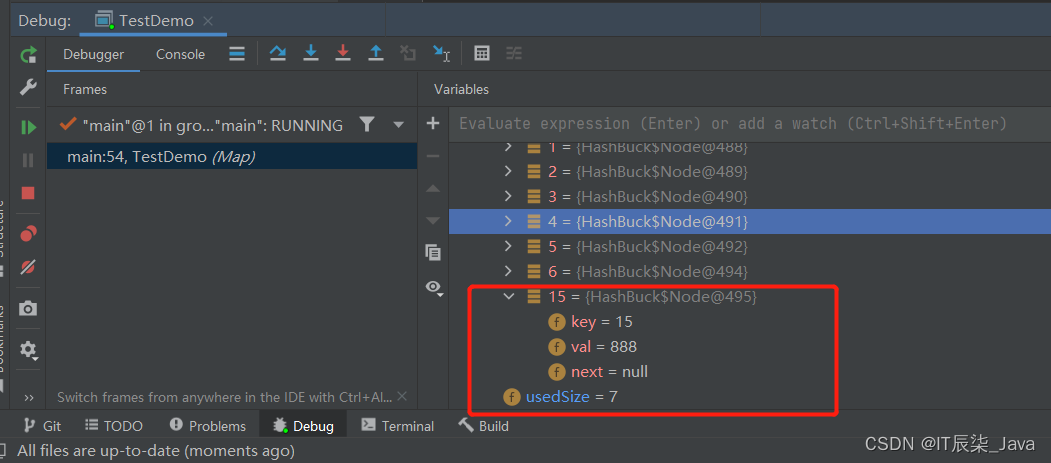🍺OK，因为这里的15 % 16 = 15；所以15应该插在index为15的位置，usedsize = 7，没有问题。

🥞4、实现get方法

``````    public int get(int key) {
int index = key % array.length;
}
}
return -1;
}``````

🥘测试：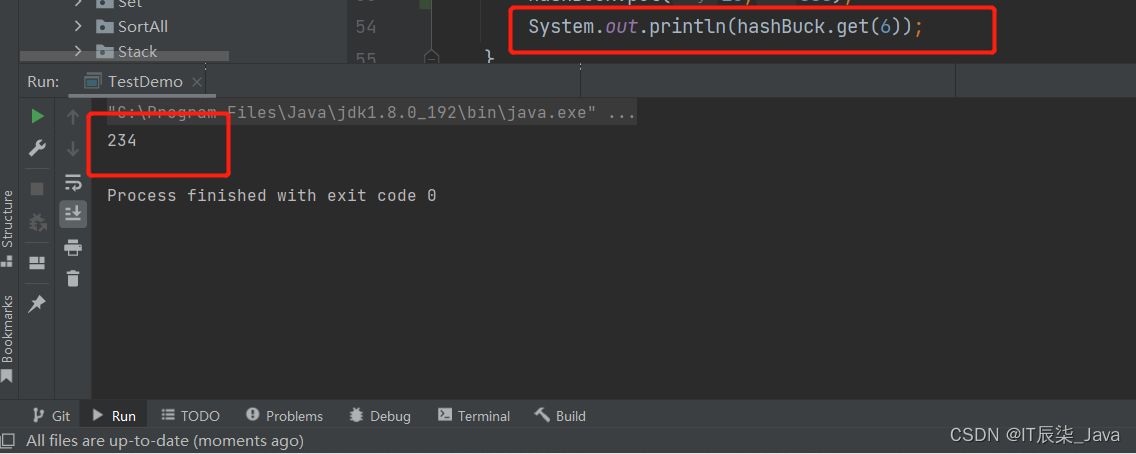### 🍏方法二(引用类型)

🍱1、内部类实现

``````    static class Node<K,V> {
public K key;
public V val;
public Node<K,V> next;

public Node(K key, V val) {
this.key = key;
this.val = val;
}
}
public Node<K,V>[] array = (Node<K,V>[])new Node;
public int usedSize;``````

🍊2、实现put方法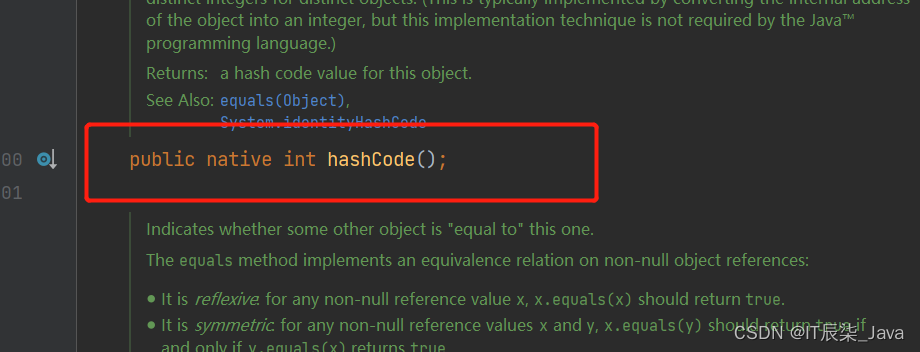🍋代码实现：

``````    public void put(K key,V val) {
int hash = key.hashCode();
int index = hash % array.length;
Node<K,V> cur = array[index];
while (cur != null) {
if(cur.key.equals(key)) {
cur.val = val;
return;
}
cur = cur.next;
}
Node<K,V> node = new Node<>(key, val);
node.next = array[index];
array[index] = node;
usedSize++;
}``````

🥙3、测试类

``````   @Override
public boolean equals(Object o) {
if (this == o) return true;
if (o == null || getClass() != o.getClass()) return false;
Person person = (Person) o;
return Objects.equals(id, person.id);
}

@Override
public int hashCode() {
return Objects.hash(id);
}
}
public class TestDemo {
public static void main(String[] args) {
Person p1 = new Person("12345");
Person p2 = new Person("12345");
System.out.println(p1.hashCode());
System.out.println(p2.hashCode());
}
}``````

🌽运行结果：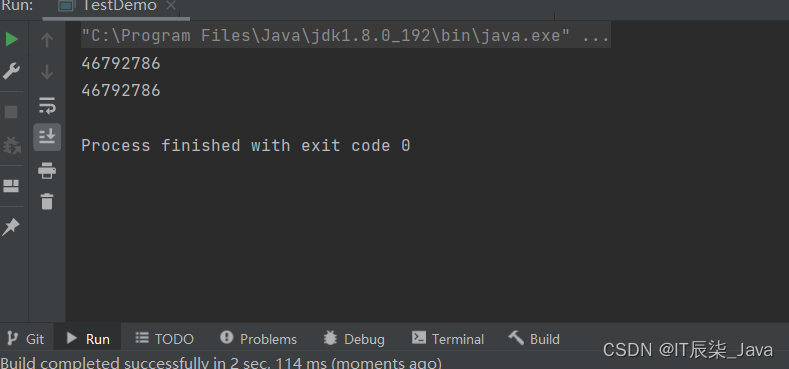🍻4、实现get方法

`````` public V get(K key) {
int hash = key.hashCode();
int index = hash % array.length;
Node<K,V> cur = array[index];
while (cur != null) {
if(cur.key.equals(key)) {
return cur.val;
}
cur = cur.next;
}
return null;
}``````

### 面试问题：

🚦1、两个对象的hashCode一样，equals一定一样吗？ 不一定

🚦2、两个对象的equals一样，hashCode一定一样吗？一定

### 原因：

🍬🍬如果两个对象hashCode()相等，它们并不一定相等。因为在散列表中，hashCode()相等，即两个键值对的哈希值相等。然而哈希值相等，并不一定能得出键值对相等，此时就出现所谓的哈希冲突场景。

🍼🍼如果两个对象相等，那么它们的hashCode()值一定相同。这里的相等是指，通过equals()比较两个对象时返回true。

### 总结：

🛥️🛥️1、hashCode() 的作用是获取哈希码，也称为散列码；它实际上是返回一个int整数。这个哈希码的作用是确定该对象在哈希表中的索引位置。hashCode() 定义在JDK的Object.java中，这就意味着Java中的任何类都包含有hashCode() 函数。

⛵⛵2、equals它的作用也是判断两个对象是否相等，如果对象重写了equals()方法，比较两个对象的内容是否相等；如果没有重写，比较两个对象的地址是否相同，价于“==”。同样的，equals()定义在JDK的Object.java中，这就意味着Java中的任何类都包含有equals()函数。